## Screenshots

•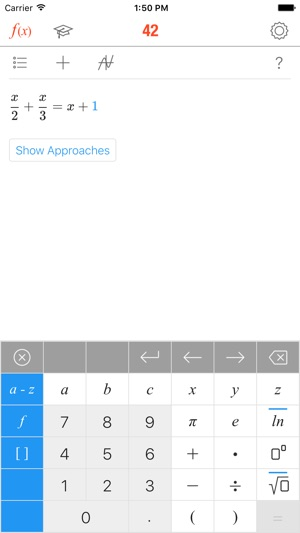•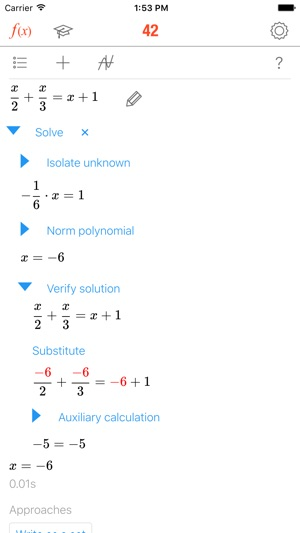•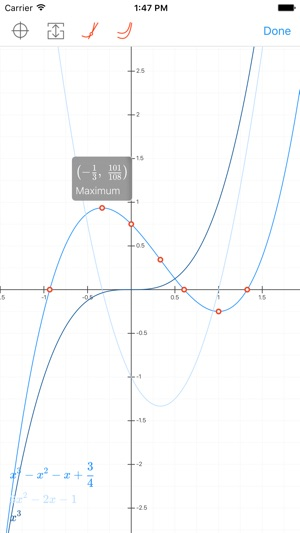•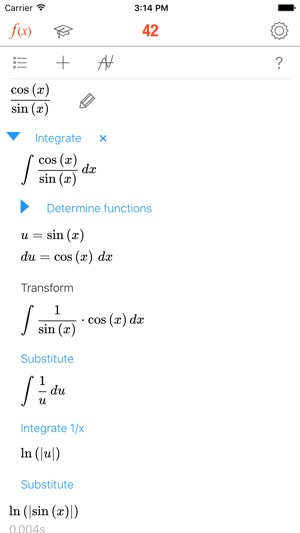•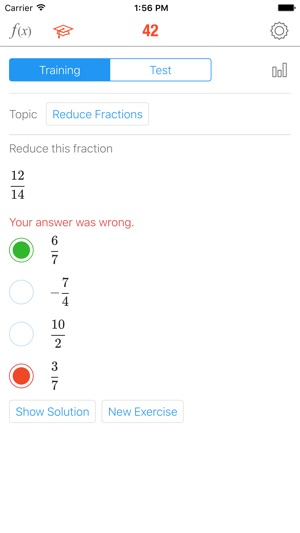## Description

With over 3.4 million downloads, MATH 42 is trusted worldwide by middle-school, high-school and college students to solve math problems, understand solutions, and get better grades. At a fraction of the cost of private tutors, MATH 42 delivers step-by-step solutions, intelligent approaches, and an Assessment Center for improving your skills.

TECHNOLOGY
• MATH 42 works without templates or pre-calculated solutions
• MATH 42 solves all problems on the fly, like a teacher at the board
• MATH 42 is a real symbolic algebra system
• MATH 42 is based on artificial intelligence

FEATURES
• Intuitive formula entry
• Calculator that instantly computes during input
• Interactive step-by-step solutions
• Suggestions on how to approach a problem
• Explanations of relevant math concepts with examples
• Interactive graphs to help visualize problems
• Assessment Center that fuels progress with tests and exercises

ASSESSMENT CENTER
• Tests and exercises created with educators and private tutors
• Training mode in which each problem can be analyzed step-by-step
• Test mode to identify strengths and weaknesses
• Results and statistics of progress over time

CAPABILITIES
Term transformation
• Simplifying and expanding
• Factorization (factoring out, applying binomial formulas)
• Transforming powers and roots
• Adding, multiplying, reducing and expanding fractions
• Computing the gcd and the lcm
• Trigonometric transformations
• Logarithmic transformations

Functions
• Roots
• Y-axis intersection
• Domain of definition
• Extremes (maximums, minimums)
• Inflexion points
• Curve sketching
• Derivation
• Fundamental derivatives
• Factor rule
• Sum rule
• Product rule
• Quotient rule
• Chain rule
• Integration
• Fundamental integrals
• Integration by substitution
• Integration by parts
• Partial fraction decomposition

Equations
• Linear equations
• Quadratic equations (completing the square, quadratic formula, pq-formula)
• Special equations of higher degree
• Linear systems of equations
• Non-linear system of equations

Matrices
• Adding matrices
• Multiplying matrices
• Transposing matrices
• Inverting matrices
• Determinants
• Characteristic polynomials
• Eigenvalues
• Eigenspaces
• Kernels
• Ranks
• Cross-product
• Gaussian elimination

## What’s New

Version 3.3.24

MATH 42 — your digital private math tutor — is completely free now!
MATH 42 now supports iPhone X.

## Ratings and Reviews

4.8 out of 5
25 Ratings

25 Ratings

Chutneys00 ,

### Incredible app

When you are stuck on a problem, this app can easily show how to do it and which method to use.

R.Piriya ,

### Impressive app

I haven't seen like this app until I downloaded this 👍🏻. It works much to impress my interest on maths😽.& I want others too, to get the massive uses from this app 👏👏👏...

glamgoldchic ,

### Thank you

Great app, refreshing the skills I learnt many years ago which I thought I'd never get back. Thank you.

## Information

Seller
Chegg, Inc.
Size
70.4 MB
Category
Education
Compatibility

Requires iOS 9.1 or later. Compatible with iPhone, iPad and iPod touch.

Languages

English

Age Rating
Rated 4+
Copyright
© 2003-2018 Chegg Inc. All rights reserved.
Price
Free

## Supports

•